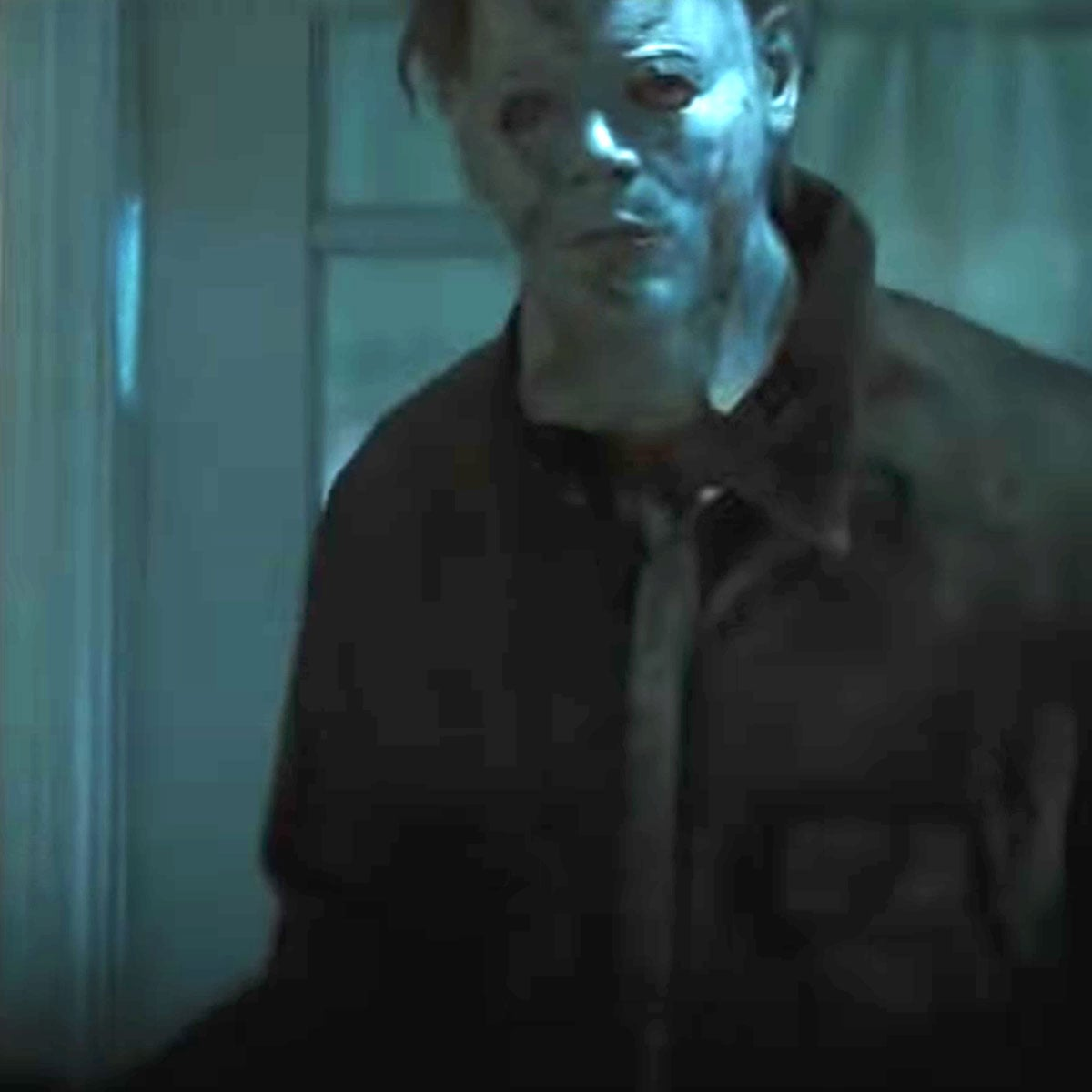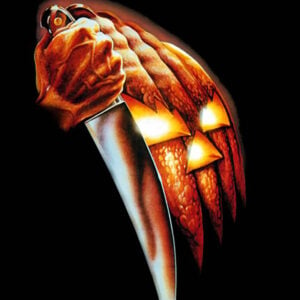# Halloween Theme (Halloween)

6 September 2020
7
03:00
-3
• ##### TEMPO
136
0
(0)

x j j x j j x j c j
x j j x j j x j c j
x j j x j j x j c j
x j j x j j x j c j
x j j x j j x j c j
x j j x j j x j c j
x j j x j j x j c j
[x6] j j x j j x j c j
[x86] j j x j j x j c j
[Z*%] H H Z H H Z H x H
Z H H Z H H Z H x H
[x6] j j x j j x j c j
[x86] j j x j j x j c j
[Z*%] H H Z H H Z H x H
Z H H Z H H Z H x H
[z5] h h z h h z h Z h
[z^5] h h z h h z h Z h
[L7\$] G G L G G L G z G
L G G L G G L G z G
[z5] h h z h h z h Z h
[z^5] h h z h h z h Z h
[L7\$] G G L G G L G z G
L G G L G G L G z G
[j2] d d j d d j d J d
[jd42] d d j d d j d J d
[jd52] d d j d d j d J d
[jd62] d d j d d j d J d
[zjd2] d d j d d j d J d
[zjd42] d d j d d j d J d
[zjd52] d d j d d j d J d
[zjd62] d d j d d j d J d
j d d j d d j d J d
x j j x j j x j c j
x j j x j j x j c j
x j j x j j x j c j
x j j x j j x j c j
[x6] j [je] x j j x j c j
[x86] j [tje] x j j x j c j
[Z*%] H [WTH] Z H H Z H x H
Z H H Z H H Z H x H
[x6] j [je] x j j x j c j
[x86] j [tje] x j j x j c j
[Z*%] H [WTH] Z H H Z H x H
Z H H Z H H Z H x H
[z5] h [wh] z h h z h Z h
[z^5] h [whE] z h h z h Z h
[L7\$] G [rQG] L G G L G z G
L G G L G G L G z G
[z5] h [wh] z h h z h Z h
[z^5] h [whE] z h h z h Z h
[L7\$] G [rQG] L G G L G z G
L G G L G G L G z G
[j2] d [d9] j d d j d J d
[jd42] d [qd9] j d d j d J d
[jd52] d [wd9] j d d j d J d
[jd62] d [ed9] j d d j d J d
[zjd2] d [d9] j d d j d J d
[zjd42] d [qd9] j d d j d J d
[zjd52] d [wd9] j d d j d J d
[zjd62] d [ed9] j d d j d J d
[zjd2] d [d9] j d d j d J d
[zjd42] d [qd9] j d d j d J d
[zjd52] d [wd9] j d d j d J d
[zjd62] d [ed9] j d d j d J d
[zjd2] d [d9] j d d j d J d
[zjd42] d [qd9] j d d j d J d
[zjd52] d [wd9] j d d j d J d
[zjd62] d [ed9] j d d j d J d
j d d j d d j d J d
x j j x j j x j c j
x j j x j j x j c j
x j j x j j x j c j
x j j x j j x j c j
x j j x j j x j c j
x j j x j j x j c j
x j j x j j x j c j
x j j x j j x j c j
x j j x j j x j c j
x j j x j j x j c j x

Rate This Music Sheet:

Average rating 0 / 5. Vote count: 0

No votes so far! Be the first to rate this music sheet.

Thank you for rating this song!

If you have any specific feedback about how to improve this music sheet, please submit this in the box below.

Halloween Theme (Halloween) is a song by John Carpenter. Use your computer keyboard to play Halloween Theme (Halloween) music sheet on Virtual Piano. This is an Intermediate song and requires a lot of practice to play well. The recommended time to play this music sheet is 03:00, as verified by Virtual Piano legend, Mark Chaimbers. The song Halloween Theme (Halloween) is classified in the genre of Songs From Movies on Virtual Piano. You can also find other similar songs using Horror.

## Other Songs By John Carpenter

•lgglgglgLglgglgglgLglgglgglgLglgglgglgLg
[4l]gglgglgLg[%l]gglgglgLg[3k]ffkffkflfkffkff[3k]flf
[4f]gglgglgLg[6l]gglgglgLg[3k]ffkffkflf[3k]ffkffkflf

Level: 4
Length: 00:32
Easy

#### John Carpenter

•k f f k f f k f l f k f f k f f k f l f k f f k f f k f l f k f f k f f k f l f [k3] f f k f f k f l f [k5] f f k f f k f l f [J%] D D J D D J D k D J D D J D D J D k D [k3] f f k f f k f l f [k5] f f k f f k f l f [J%] D D J D D J D k D [J6] D D J D D J D k D [j2] d d j d d j d J d [j4] d d j d d j d J d [H!] S S H S S H S j S H S S H S S H S j S [j2] d d j d d j d J d [j4] d d j d d j d J d [H!] S S H S S H S j S H S S H S S H S j S [f6] p p f p p f p g p [f1] p p f p p f p g p [f2] p p f p p f p g p [f3] p p f p p f p g p [f6] p p f p p f p g p [f1] p p f p p f p g p [f2] p p f p p f p g p [f3] p p f p p f p g p f p p f p p f p g p f p p f p p f p g p
Level: 4
Length: 01:09
Easy

## Related

•[8w]|o p [8ws]|p f [8w]|s s [8w]|| [8w]|o p [8ws]|p f [8w]|s s [8w]|h h [9w]||[9w]|| [9wh] h|h [9q] g|g [8w] f|f [8w]|| [8w]|o p [8ws]|p f [8w]|s s [8w]|| [8w]|o p [8ws]|p f [8w]|s s [8w]|h h [9w]||[9w]|| [9wh] h|h [9w] g|g [8w] f|f [8w]|| [qe]||[qej]| hg[9wh]||[7w]|f g [8wh] h| [7wh]| s [60s]|||| [qe]||[qej]| hg[9wh]||[7w]|f g [8wh] h| [7wh]| s [60s]|||| [8q]||[8qp] s|s [9w]|o o [7wf]|s s [80w] [8w]|o p [8ws]|p f [8w]|s s [8w]|| [8w]|o p [8ws]|p f [8w]|s s [8w]|h h [9w]||[9w]|| [9wh] h|h [9q] g|g [8w] f|f [8w]|| [8w]|o p [8ws]|p f [8w]|s s [8w]|| [8w]|o p [8ws]|p f [8w]|s s [8w]|h h [9w]||[9w]|| [9wh] h|h [9w] g|g [8w] f|f [8w]|| [qe]||[qej]| hg[9wh]||[7w]|f g [8wh] h| [7wh]| s [60s]|||| [qe]||[qej]| hg[9wh]||[7w]|f g [8wh] h| [7wh]| s [60s]|||| [8q]||[8qp] s|s [9w]|o o [7wf]|s s [80w]
Level: 5
Length: 02:30
Intermediate

#### Creedence Clearwater Revival

•5 [u8] 5 [y9] 5 [t0] 5 [w3] 7 [o0] 7 [QI] 7 [wu] 7 [e4] 8 [uq] 8 [yw] 8 [te] 8  5 [w8] 5 [t0] 5 [y7] w [t60] 0 [se] 0 [ra] 0 [ut] 0 [w3] 7 [o0] 7 [QI] 7 [wr] 7  5 [u8] 5 [y9] 5 [t0] 5 [te5] 5 [wr9] 5 [e8] 5 [w7] 5 [q2] 6 [i9] 6 [u0] 6 [qe] 6 [q^] 4 [uo^] 4 [yi8] 4 [qE9] 4 [qe2] 6 [i9] 6 [u2] [y6] [yu9] [i6] [tp5] 2 [ro5] 2 [ie5] 2 [wo7] 2 [w10] 5 [u8] 5 y 5 [w0] 5 [w30] 7 [o0] 7 I 7 [wr] 7 [w10] 5 [u8] 5 y 5 [w0] 5 [w30] 7 [o0] 7 I 7 [wr] 7 [qe2] 6 [o9] 6 [u2] 6 [qe] 6 [qe5] 2 [o6] 2 [wr5] 2 [oh7] 2 [sl1] 5 [ka0] w [sl1] 5 [uf8] w [oh3] 7 Q w 3 7 [oh0] w [pj4] 8 [woh] e [pj4] 8 [tsq] e [uf1] 5 8 w [yd7] 5 [ka9] w [sl6] 6 [ka9] 6 [sl0] 6 [uf8] 6 [oh3] 7 Q 7 w 7 [h0] 7 [ig2] 6 [uf9] 6 [yd2] 6 [tsq] 6 [uf5] 5 0 5 5 5 0 5 [yd5] 5 7 5 [oha9] 5 [slf1] 5 [kda0] w [slf1] 5 [uof0] w [oha3] 7 Q w 3 7 [oha0] w [spj4] 8 [woha] e [spj4] 8 [tsqp] e [uof1] 5 8 w [yod7] 5 [kda9] w [slf6] 6 [kda9] 6 [slf0] 6 [usf8] 6 [oha3] 7 Q 7 w 7 [oh0] 7 [ig2] 6 [uf0] q [yd2] 6 [ts9] q [ig^] ^ [uqf] y [yd^] ^ [tsq] E [ig5] 5 [uf9] w [yd5] 5 w [ts] [us1] 8 w t w|d| [oa3] 7 0 r 0|o| [us1] 8 w t w|d| [oa3] 7 0 r 0|o| [us1] 8 w t w|d| [oa3] 7 0 r 0|o| [us1] 5 [u8] 5 [y9] 5 [t0] 5 [w3] 7 [o0] 7 [QI] 7 [wu] 7 [e4] 8 [uq] 8 [yw] 8 [te] 8  5 [w8] 5 [t0] 5 [y7] w [t60] 0 [se] 0 [ra] 0 [ut] 0 [w3] 7 [o0] 7 [QI] 7 [wr] 7  5 [u8] 5 [y9] 5 [t0] 5 [te5] 5 [wr9] 5 [e8] 5 [w7] 5 [q2] 6 [i9] 6 [u0] 6 [qe] 6 [q^] 4 [uo^] 4 [yi8] 4 [qE9] 4 [qe2] 6 [i9] 6 [u2] [y6] [yu9] [i6] [tp5] 2 [ro5] 2 [ie5] 2 [wo7] 2 [w10] 5 [u8] 5 y 5 [w0] 5 [w30] 7 [o0] 7 I 7 [wr] 7 [qe2] 6 [p9] 6 [o0] 6 [i9] 6 [wr5] 9 [wo] 9 [ie] 9 [wr] 9 [qe2] 6 [i9] 6 [u0] 6 [qe] 6 [w5] 2 [u5] 2 [w7] 2 [q5] 2 
Level: 6
Length: 03:05
Intermediate

#### Hans Zimmer

•[6p] [2qg] [6p] [0f] [6p] [2qg] [wh] [0f] [6p] [wh] [qg] [0f] [d9] [1580f] [48qej] [ej] [EJ] [wh] [s8] [s8] [48qej] [EJ] [wh] [d9] [5wEJ] [ej] [wh] [qg] [60f] [6p] [26qg] [6p] [0f] [6p] [2qg] [wh] [0f] [6p] [29wh] [qg] [0f] [d9] [1580f] [s8] [4qej] [EJ] [wh] [s8] [4qej] [EJ] [8wh] [d9] [59wEJ] [ej] [0wh] [qg] [d269] 2 p[269f] g d 9 g [60pg] f p p [260f] g d q g [6psg] f p [^qaf] g d [^q] j [59wdj] h [59wdP] f [qdg^P] d [60pf] s [269ipd] p [qf^P] g d j [jdw59] h d [fx] [gcl^] [dz] [jbz6] [hv] [fxh] [gc] [2dgjc]
Level: 5
Intermediate